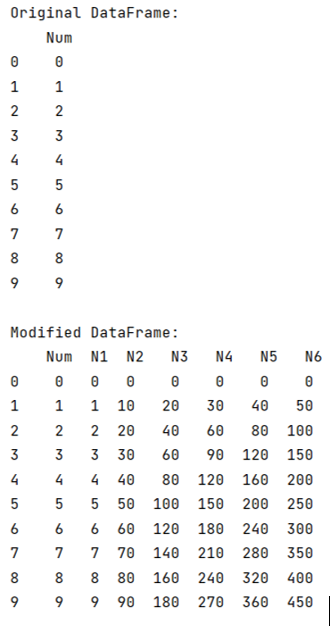# How to apply Pandas function to column to create multiple new columns?

Given a Pandas DataFrame, we have to apply pandas function to columns to create multiple new columns. By Pranit Sharma Last updated : September 20, 2023

Columns are the different fields that contain their particular values when we create a DataFrame. We can perform certain operations on both rows & column values. Here, we are going to learn how to apply a function to a column to create multiple columns.

## Problem statement

Given a Pandas DataFrame, we have to apply pandas function to columns to create multiple new columns.

## Applying Pandas function to column to create multiple new columns

For this purpose, we are going to define a function that will return multiple values, we will then zip these multiple values and map them into multiple columns in a DataFrame.

Here, we are going to use zip() function, below is the syntax:

```zip('object_1','object_2',.....,'object_n')
```

The zip() function is used to return zipped objects, it contains an iterator, and one by one it passes a value and pairs them.

Also, we are going to map function, which is used to map multiple values, here we will use the map function to call our defined function for getting multiple values the multiple values and the column of DataFrame will be joined together by zip() function.

Note

To work with pandas, we need to import pandas package first, below is the syntax:

```import pandas as pd
```

Let us understand with the help of an example.

## Python program to apply Pandas function to column to create multiple new columns

```# Importing Pandas package
import pandas as pd

# Create a dictionary
d= {'Num': [ i for i in range(10)]}

# Create DataFrame
df1 = pd.DataFrame(d)

# Display DataFrame
print("Original DataFrame:\n",df1,"\n")

# Defining a function
def function(n):
return n, n*10, n*20, n*30, n*40, n*50

# Making 6 new columns and zipping all the 6 values
# coming from function into these columns
df1['N1'],df1['N2'],df1['N3'],df1['N4'],df1['N5'],df1['N6'] = zip(*df1['Num'].map(function))

# Display modified DataFrame
print("Modified DataFrame:\n",df1)
```

### Output

The output of the above program is: